Explain how find the Slope for a line passing through (-2, 6) and (4.-3) What is the slope passing through (-2-,6) and (4

Question

Explain how find the Slope for a line passing through (-2, 6) and (4.-3)

What is the slope passing through (-2-,6) and (4.-3)

in progress 0
6 months 2021-08-02T15:18:22+00:00 1 Answers 4 views 0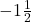Step-by-step explanation:

Slope is calculated using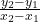.

We’ll just substitute in our points.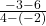Simplify.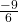Simplify…..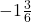More…And we’re done!